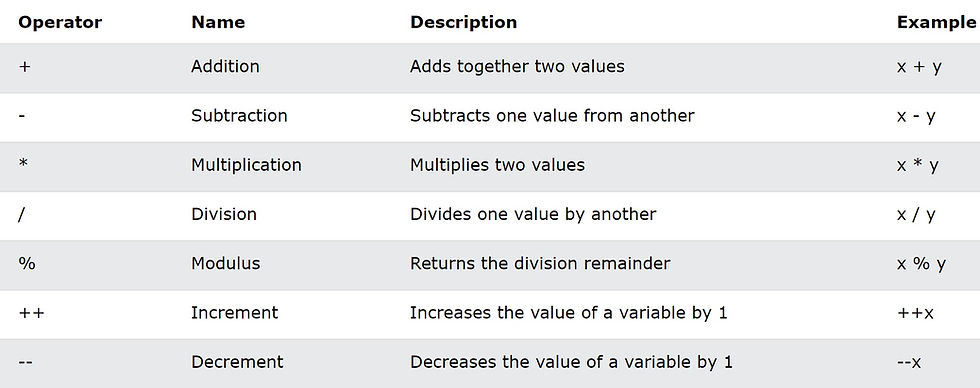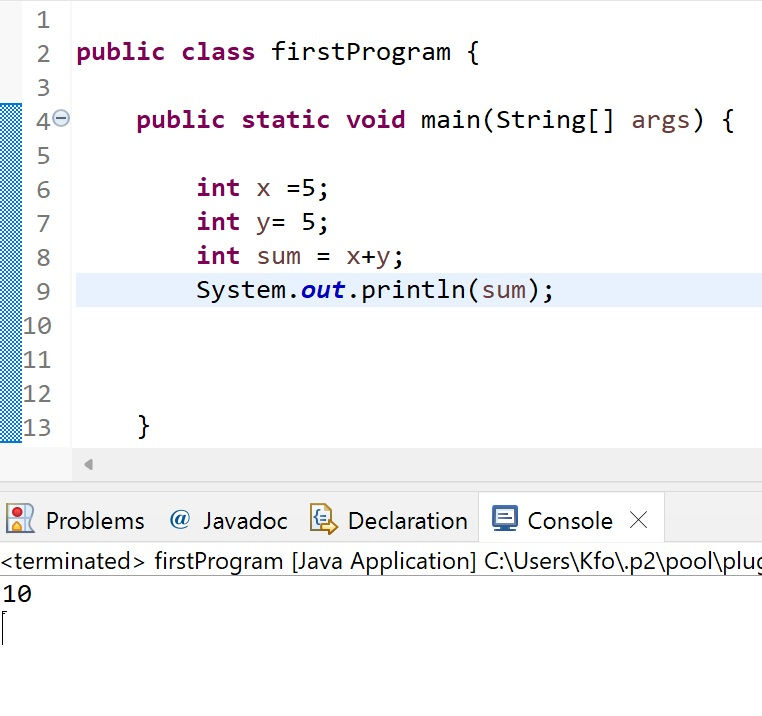•A.razaq

# T7- Java operators

in java, Operators are used to perform operations on variables and values to preform calculations and algebraic expressions

operators are :lets test them one by one

```int x =5;
int y= 5;
int sum = x+y;
System.out.println(sum);```

now the output will be :

`10`we can also write text before placing our variable value by writing out text inside a double quotation marks then + to add the value of x to our message

meaning

System.out.println(" display a specific message here " + our variable which is x );

the code will be

```int x= 5+5;
System.out.println(" the result of x is = " + x);```

the output will be :

` the result of x is = 10`-------------

assignment operators

we use assignment operators to assign a value to a variable or add a value to a variable as wellif we want to assign the value 5 to the variable x we simply like in the previous tutorials do the following

`int x =5;`

we can assign a variable and value to it by using the += operator

```int x =10;
x += 1;
System.out.println(x);```

now the output will beso the expression above is just exactly as doing this

```int x =10;
x = x + 1;
System.out.println(x);```we can also increment and decrement values by using the operator ++ or --

```int x =10 ;
x++;
System.out.println(x);```

this will increment the value of x by 1

the output will be

`11`we can do the same for decrementing as well

```int x =10 ;
x--;
System.out.println(" the value of x after decrement is : " +x);```

the output will be :

```
the value of x after decrement is : 9```